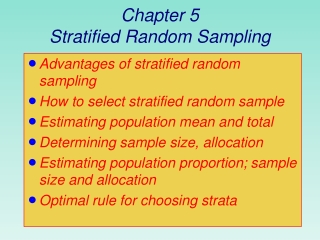# Chapter 5 Stratified Random Sampling - PowerPoint PPT PresentationDownload PresentationChapter 5 Stratified Random Sampling

Chapter 5 Stratified Random Sampling
Download Presentation## Chapter 5 Stratified Random Sampling

- - - - - - - - - - - - - - - - - - - - - - - - - - - E N D - - - - - - - - - - - - - - - - - - - - - - - - - - -
##### Presentation Transcript

1. Chapter 5Stratified Random Sampling • Advantages of stratified random sampling • How to select stratified random sample • Estimating population mean and total • Determining sample size, allocation • Estimating population proportion; sample size and allocation • Optimal rule for choosing strata

2. Stratified Random Sampling • The ultimate function of stratification is to organize the population into homogeneous subsets and to select a SRS of the appropriate size from each stratum.

3. Warmup You are doing a project to study grade inflation in the STEM disciplines. A quick internet search shows that average GPA differs by STEM major. In addition, overall GPA differs by school, so you don’t want to limit your sample to “convenient” schools.

4. Warmup (cont.) • Data from the National Center for Education Statistics results in the following percentages of majors among STEM disciplines: • Computer Science24% • Engineering 21% • Bio/Life Sciences 17% • Math/Stat 15% • Technology 12% • Chemistry 7% • Physics 4% • Approximately 15 million students are in public colleges and 5 million are in private colleges. • Suppose you would like to use a sample size of n = 1,000 students.

5. Stratified Random Sampling • Often-used option b/c … • May produce smaller BOE than SRS of same size • Cost per observation may be reduced • Obtain estimates of population parameters for subgroups • Useful when the population is heterogeneous and it is possible to establish strata which are reasonably homogeneous within each stratum

6. Chapter 5Stratified Random Sampling Improved Sampling Designs with Auxiliary Information Chapter 5 Stratified Random Sampling Chapter 6 Ratio and Regression Estimators

7. Stratified Random Sampling: Notation

8. Warmup (cont.) • Selecting the stratified random sample. • Recall: • approximately 15 million students are in public colleges and 5 million are in private colleges. • Suppose you would like to use a sample size of n = 1,000 students.

9. Stratified Random Sampling

10. Stratified Random Sampling: Estimate of Mean 

11. Stratified Random Sampling: Estimate of Mean , BOE

12. Stratified Random Sampling: Estimate of Population Total 

13. Stratified Random Sampling: BOEfor Mean  and Total , t distribution • When stratum sample sizes are small, can use t dist.

14. Degrees of Freedom(worksheet cont.)

15. Compare BOE in Stratified Random Sample and SRS (worksheet cont.) Strat. random sample has more precision

16. Approx. Sample Size to Estimate µ

17. Approx. Sample Size to Estimate 

18. Summary: Approx. Sample Size to Estimate µ,

19. Example: Sample Size to Estimate µ (worksheet cont.)

20. Example: Sample Size to Estimate  (worksheet cont.)

21. 5.5 Allocation of the Sample • Objective: obtain estimators with small variance at lowest cost. • Allocation affected by 3 factors: • Total number of elements in each stratum • Variability in each stratum • Cost per observation in each stratum

22. 5.5 Allocation of the Sample: Proportional Allocation • If don’t have variability and cost information for the strata, can use proportional allocation. In general this is not the optimum choice for the stratum sample sizes.

23. Directly proportional to stratum size and stratum variability

24. Worksheet 12

25. Directly proportional to stratum size and stratum variability Inversely proportional to stratum cost/obs

26. Worksheet 13